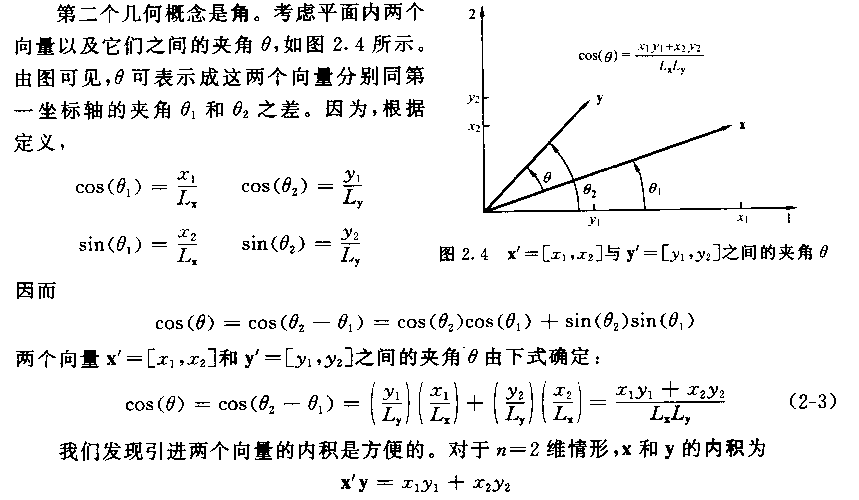# 点乘与叉乘

12 篇文章 0 订阅
﻿﻿

## 几何学定义与例子$\mathbf{a}\cdot \mathbf{b} = \sum_{i=1}^n a_ib_i = a_1b_1 + a_2b_2 + \cdots + a_nb_n$$\begin{bmatrix}1&3&-5\end{bmatrix} \cdot \begin{bmatrix}4&-2&-1\end{bmatrix} = (1)(4) + (3)(-2) + (-5)(-1) = 3$$\mathbf{a} \cdot \mathbf{b} = \mathbf{a} \mathbf{b}^T$$\begin{bmatrix} 1&3&-5\end{bmatrix}\begin{bmatrix} 4\\-2\\-1\end{bmatrix} = \begin{bmatrix} 3\end{bmatrix}$

## 几何解释$\mathbf{a} \cdot \mathbf{b} = |\mathbf{a}| \, |\mathbf{b}| \cos \theta \;$,$\cos{\theta} = \frac{\mathbf{a \cdot b}}{|\mathbf{a}| \, |\mathbf{b}|}$$\left \langle \mathbf{a}, \mathbf{b} \right \rangle = \sum_{i=1}^n a_ib_i$09-18
02-2513万+03-173566
12-18987
02-06635
03-18269
06-25145
03-14780
08-071348
02-063万+
09-0262万+
02-227万+
08-203万+
03-231万+
11-0611万+
05-131247
11-204755
08-061万+
05-241万+点击重新获取扫码支付余额充值